Question

# A student has six different math books, four different history books, and seven different science books....

A student has six different math books, four different history books, and seven different science books. In how many different ways can they be arranged on a shelf if books in the same category are kept next to one another? Show the calculations that lead to your answer.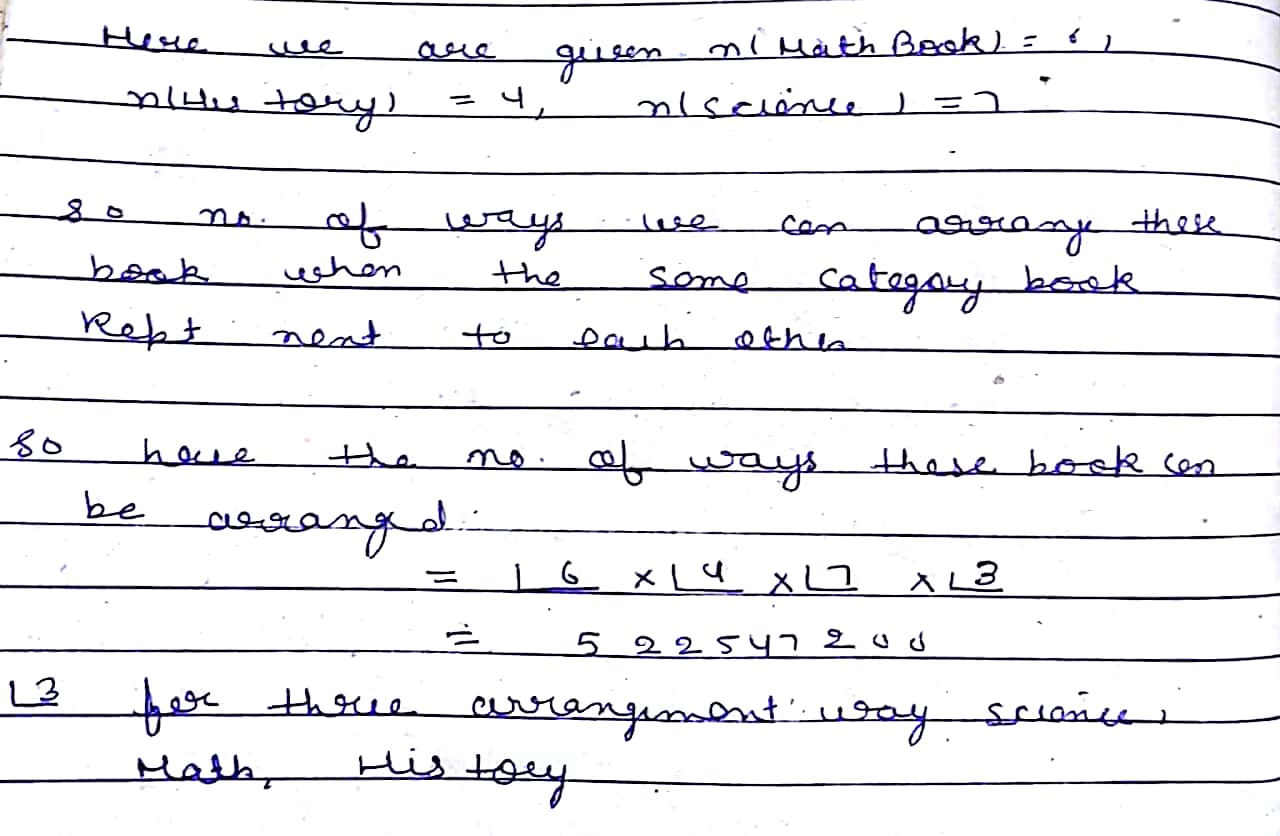#### Earn Coins

Coins can be redeemed for fabulous gifts.

Similar Homework Help Questions
• ### you have 2math books, 3history books and 7science books. how many ways are there to arrange...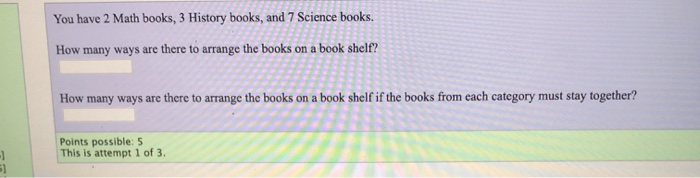you have 2math books, 3history books and 7science books. how many ways are there to arrange the books on a book shelf? how many ways are there to arrange the books on a book shelf if the books from each category must stay together? You have 2 Math books, 3 History books, and 7 Science books. How many ways are there to arrange the books on a book shelf? How many ways are there to arrange the books on a...

• ### Kelly has different books to arrange on a shelf; 2 blue, 4 green, and 3 red....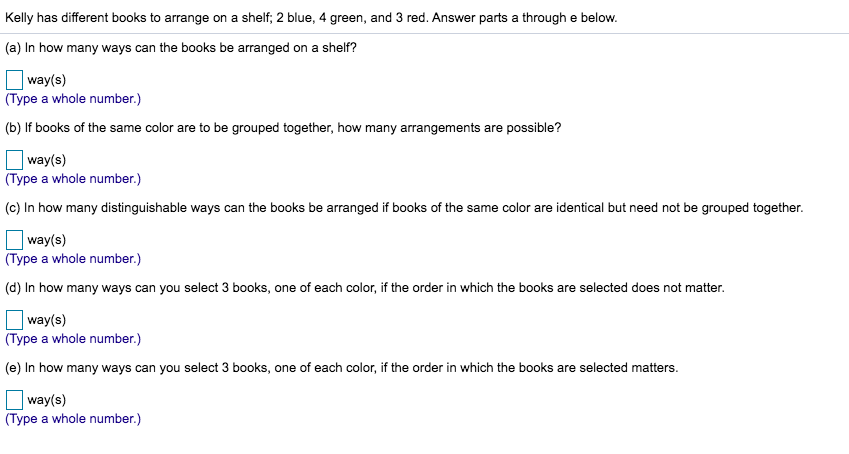Kelly has different books to arrange on a shelf; 2 blue, 4 green, and 3 red. Answer parts a through e below. (a) In how many ways can the books be arranged on a shelf? way(s) (Type a whole number.) (b) If books of the same color are to be grouped together, how many arrangements are possible? way(s) (Type a whole number.) (c) In how many distinguishable ways can the books be arranged if books of the same color are...

• ### 6. A box contains 8 novels and 7 math books going to loan 6 books to...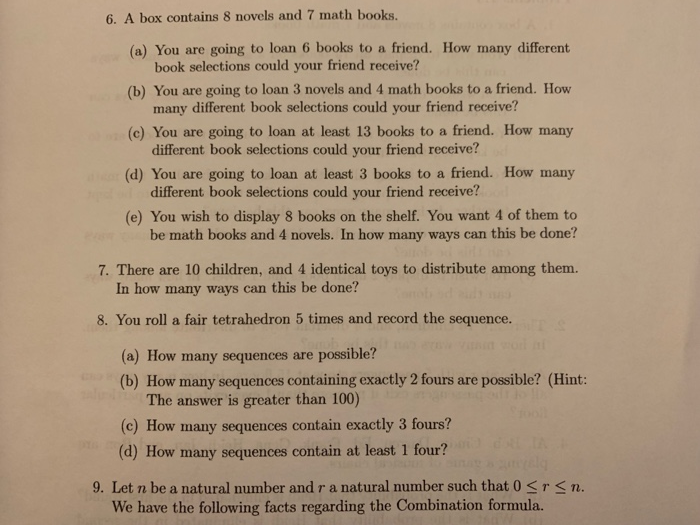6. A box contains 8 novels and 7 math books going to loan 6 books to a friend. How many different (a) You are book selections could your friend receive? going to loan 3 novels and 4 math books to a friend. How (b) You are many different book selections could your friend receive? (c) You are going to loan at least 13 books to a friend. How many different book selections could your friend receive? going to loan at...

• ### 1. Assume that there are an arbitrarily large number of copies of ten different discrete math books (which we will call titles) for cmsc250. Each student must choose one title as the course text book...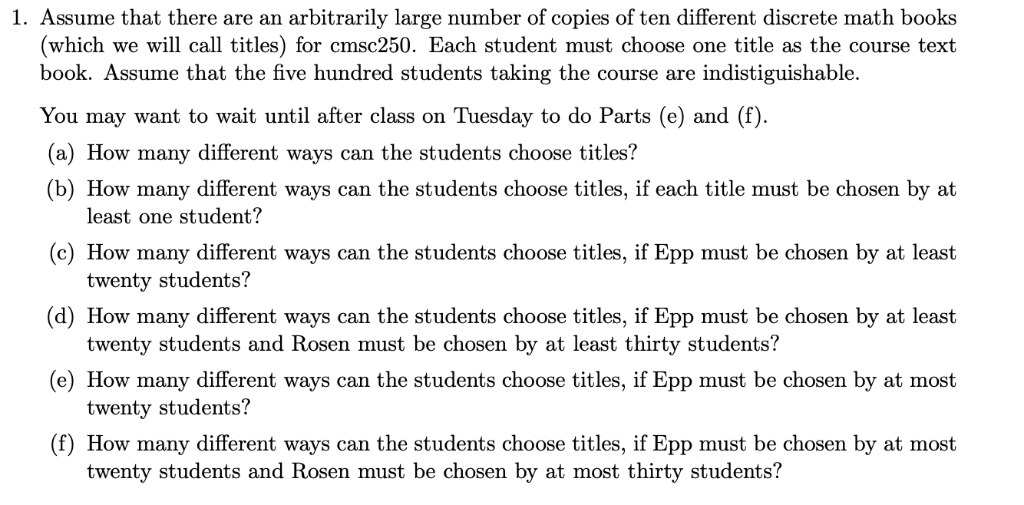1. Assume that there are an arbitrarily large number of copies of ten different discrete math books (which we will call titles) for cmsc250. Each student must choose one title as the course text book. Assume that the five hundred students taking the course are indistiguishable. You may want to wait until after class on Tuesday to do Parts (e) and (f) (a) How many different ways can the students choose titles? (b) How many different ways can the students...

• ### 6 different books are to be distributed among the 10 students in a class. A student...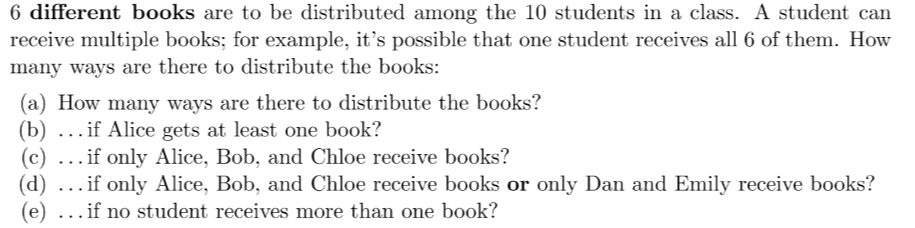6 different books are to be distributed among the 10 students in a class. A student can receive multiple books; for example, it's possible that one student receives all 6 of them. How many ways are there to distribute the books: (a) How many ways are there to distribute the books? (b) ... if Alice gets at least one book? (c) ... if only Alice, Bob, and Chloe receive books? (d) ... if only Alice, Bob, and Chloe receive books...

• ### [Computer Science, CS2203 - Databases 1] “A student can attend six courses, each with a different...

[Computer Science, CS2203 - Databases 1] “A student can attend six courses, each with a different instructor. Each instructor teaches at least 3 courses.” What is the type of relationship between students and instructors? Select one: a. one-to-one b. one-to-many c. many-to-many d. None of the above

• ### Josephine has eight chemistry books, three history books, and ten statistics books. She wants to choose...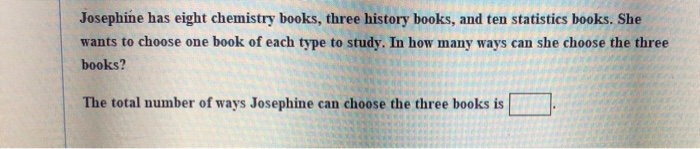Josephine has eight chemistry books, three history books, and ten statistics books. She wants to choose one book of each type to study. In how many ways can she choose the three books? The total number of ways Josephine can choose the three books is

• ### 4. A student must answer seven questions on an exam that contains twelve questions. a. How...

4. A student must answer seven questions on an exam that contains twelve questions. a. How many ways can the student do this? b. How many ways are there if the student must answer the first and last question? PLEASE SHOW WORK

• ### 1. A box contains 16 books, 9 paperbacks and 7 hardcovers. Each book has a different...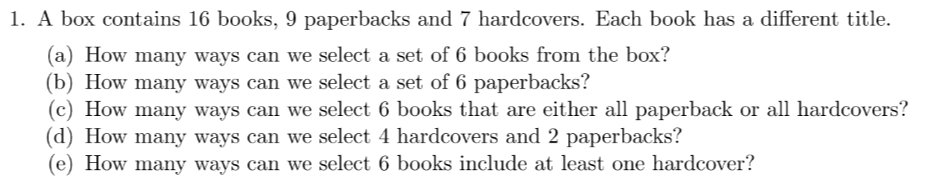1. A box contains 16 books, 9 paperbacks and 7 hardcovers. Each book has a different title. (a) How many ways can we select a set of 6 books from the box? (b) How many ways can we select a set of 6 paperbacks? (c) How many ways can we select 6 books that are either all paperback or all hardcovers? (d) How many ways can we select 4 hardcovers and 2 paperbacks? (e) How many ways can we select...

• ### I have nine different programming textbooks on my bookshelf, five C++ and four Java. In how many ways can I arrange the...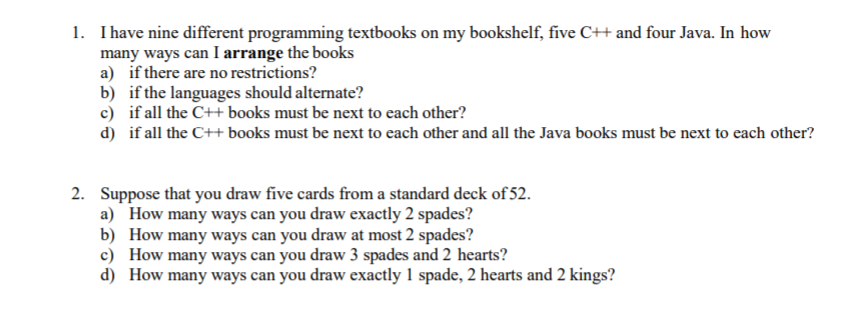I have nine different programming textbooks on my bookshelf, five C++ and four Java. In how many ways can I arrange the books a) if there are no restrictions? b) ifthe languages should alternate? c) if all the C++books must be next to each other? d) if all the C++books must be next to each other and all the Java books must be next to each other? 1. 2. Suppose that you draw five cards from a standard deck of...